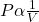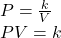Question

Pressure and volume changes at a constant temperature can be calculated using

1.RobertKer

Option A, Boyle’s law

Explanation:

The complete question is

Pressure and volume changes at a constant temperature can be calculated using

a. Boyle’s law. c. Kelvin’s law.

b. Charles’s law. d. Dalton’s law.

Solution

In Boyle’s law, the gas is assumed to be ideal gas and at constant  temperature. With these two conditions fixed, Boyle’s established that volume of gas varies inversely with the absolute pressure.

The basic mathematical representation of this phenomenon is as follows –ORWhere P is the pressure of ideal gas, V is the volume and k is the constant of proportionality.

Hence, option A is correct